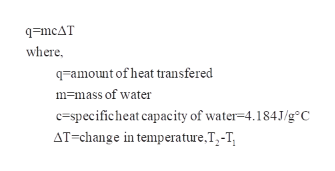# In a calorimeter, 100.0 g of water, initially at 21.8 *C, is mixed with 0.300 moles of LiOH. After dissolving, the temperature of the water is 38.7 *C. What was the heat of dissolution (dHdiss) of LiOH in kJ/mole (3 sig figs)

Question
6 views

In a calorimeter, 100.0 g of water, initially at 21.8 *C, is mixed with 0.300 moles of LiOH. After dissolving, the temperature of the water is 38.7 *C. What was the heat of dissolution (dHdiss) of LiOH in kJ/mole (3 sig figs)

check_circle

Step 1

Given:

Mass of water=100 g

Moles of LiOH=0.300 moles

Initial temperature=21.8oC

Final temperature=38.7oC

Step 2

To calculate heat of dissolution:

Heat of dissolution also called enthalpy of dissolution, the equation is given ashelp_outlineImage Transcriptioncloseq=mcAT where, q-amount of heat transfered m=mass of water c=specificheat capacity of water=4.184J/g°C AT=change in temperature,T,-T, fullscreen
Step 3

First we have to calculate the amount of heat transfer...

### Want to see the full answer?

See Solution

#### Want to see this answer and more?

Solutions are written by subject experts who are available 24/7. Questions are typically answered within 1 hour.*

See Solution
*Response times may vary by subject and question.
Tagged in